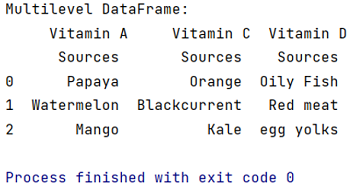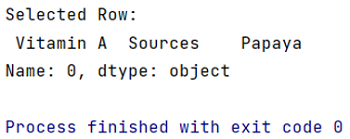# How to select rows in pandas MultiIndex DataFrame?

Given a pandas MultiIndex DataFrame, we have to select rows.
Submitted by Pranit Sharma, on May 27, 2022

Columns are the different fields which contains their particular values when we create a DataFrame. We can perform certain operations on both rows & column values. In this article, we are going to learn how to drop a level from a multi-level column index.

Multilevel indexing is a type of indexing which include different levels of indexes or simply multiple indexes. The DataFrame is classified under multiple indexes and the topmost index layer is presented as level 0 of multilevel index followed by level 1, level 2 and so on.

To understand how to select a row from a multiindex DataFrame, we first need to create a multilevel index DataFrame.

To work with pandas, we need to import pandas package first, below is the syntax:

```import pandas as pd
```

Example:

```# Importing pandas package
import pandas as pd

# Creating multilevel index
index = pd.MultiIndex.from_tuples([('Vitamin A','Sources'),
('Vitamin C', 'Sources'),
('Vitamin D','Sources')])

# Creating a multilevel index DataFrame with
# columns = multilevel indexes
df = pd.DataFrame([['Papaya','Orange','Oily Fish'],
['Watermelon','Blackcurrent','Red meat'],
['Mango','Kale','egg yolks']], columns=index)

# Display multilevel DataFrame
print("Multilevel DataFrame:\n",df)
```

Output:Now we will select a row from this Multiindex DataFrame. We will use DataFrame.loc() for the purpose of selecting a row.

```# Selecting row from this
# multiindex DataFrame
result = df.loc[0,['Vitamin A']]

print("Selected Row:\n",result)
```

Output:What's New (MCQs)

Top Interview Coding Problems/Challenges!

IncludeHelp's Blogs

Languages: » C » C++ » C++ STL » Java » Data Structure » C#.Net » Android » Kotlin » SQL
Web Technologies: » PHP » Python » JavaScript » CSS » Ajax » Node.js » Web programming/HTML
Solved programs: » C » C++ » DS » Java » C#
Aptitude que. & ans.: » C » C++ » Java » DBMS
Interview que. & ans.: » C » Embedded C » Java » SEO » HR
CS Subjects: » CS Basics » O.S. » Networks » DBMS » Embedded Systems » Cloud Computing
» Machine learning » CS Organizations » Linux » DOS
More: » Articles » Puzzles » News/Updates